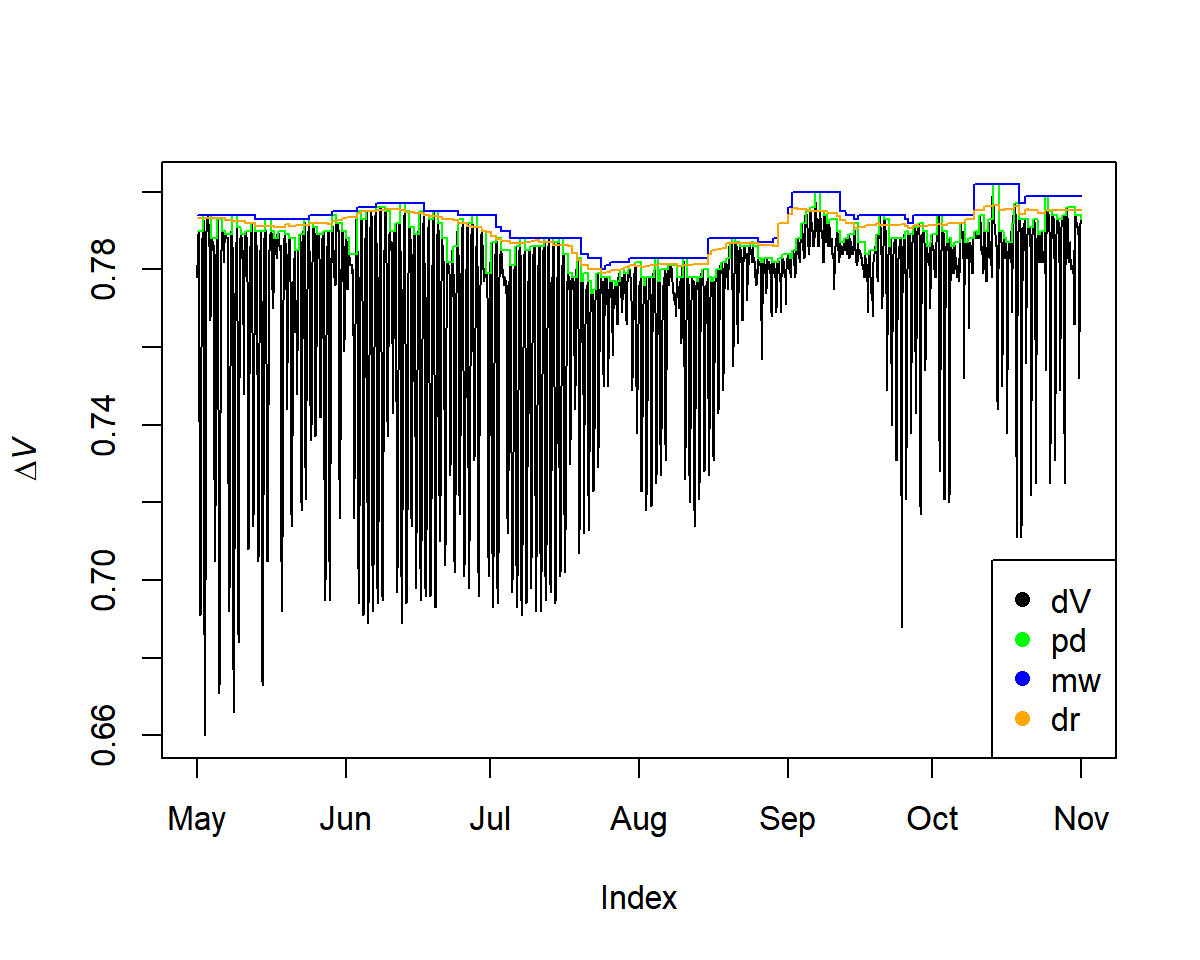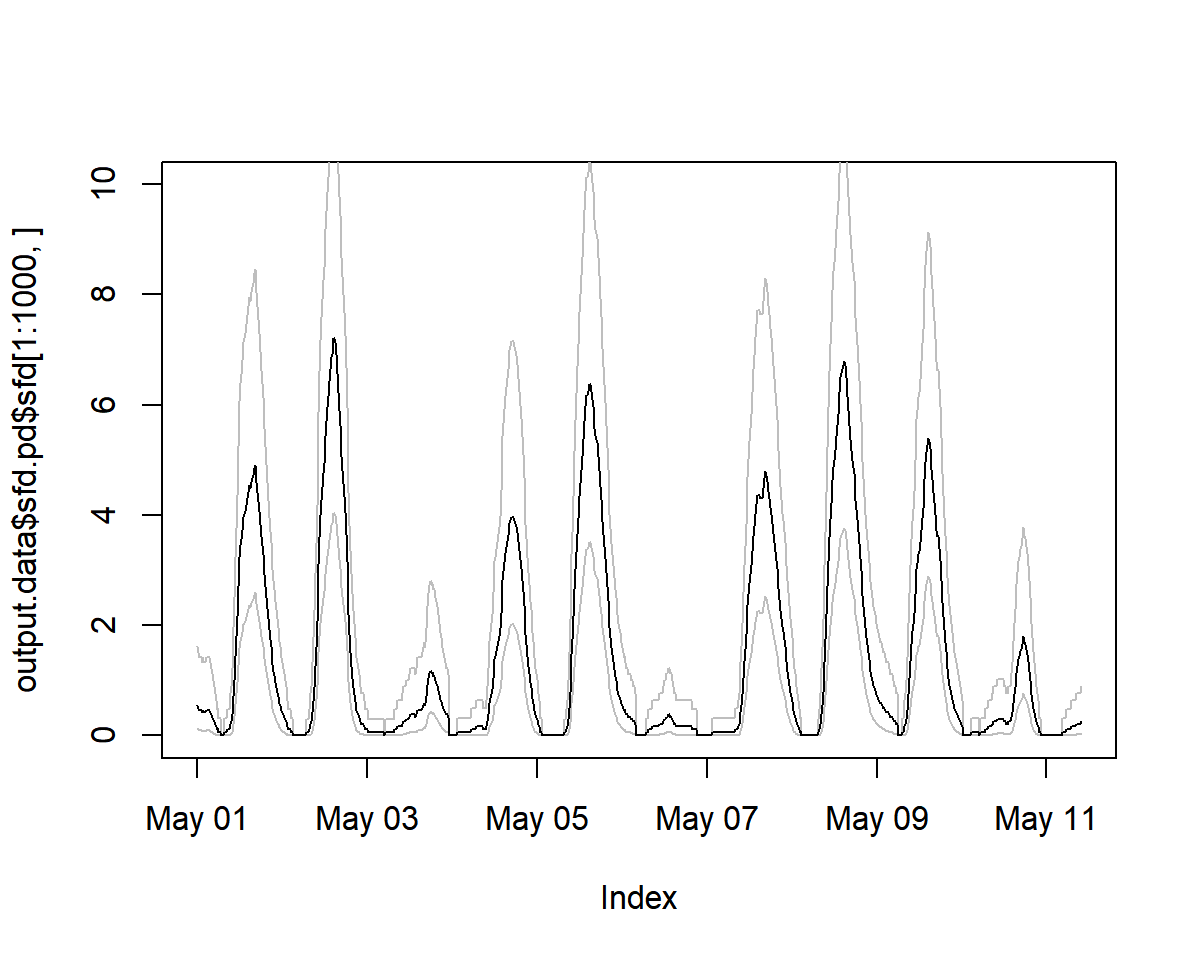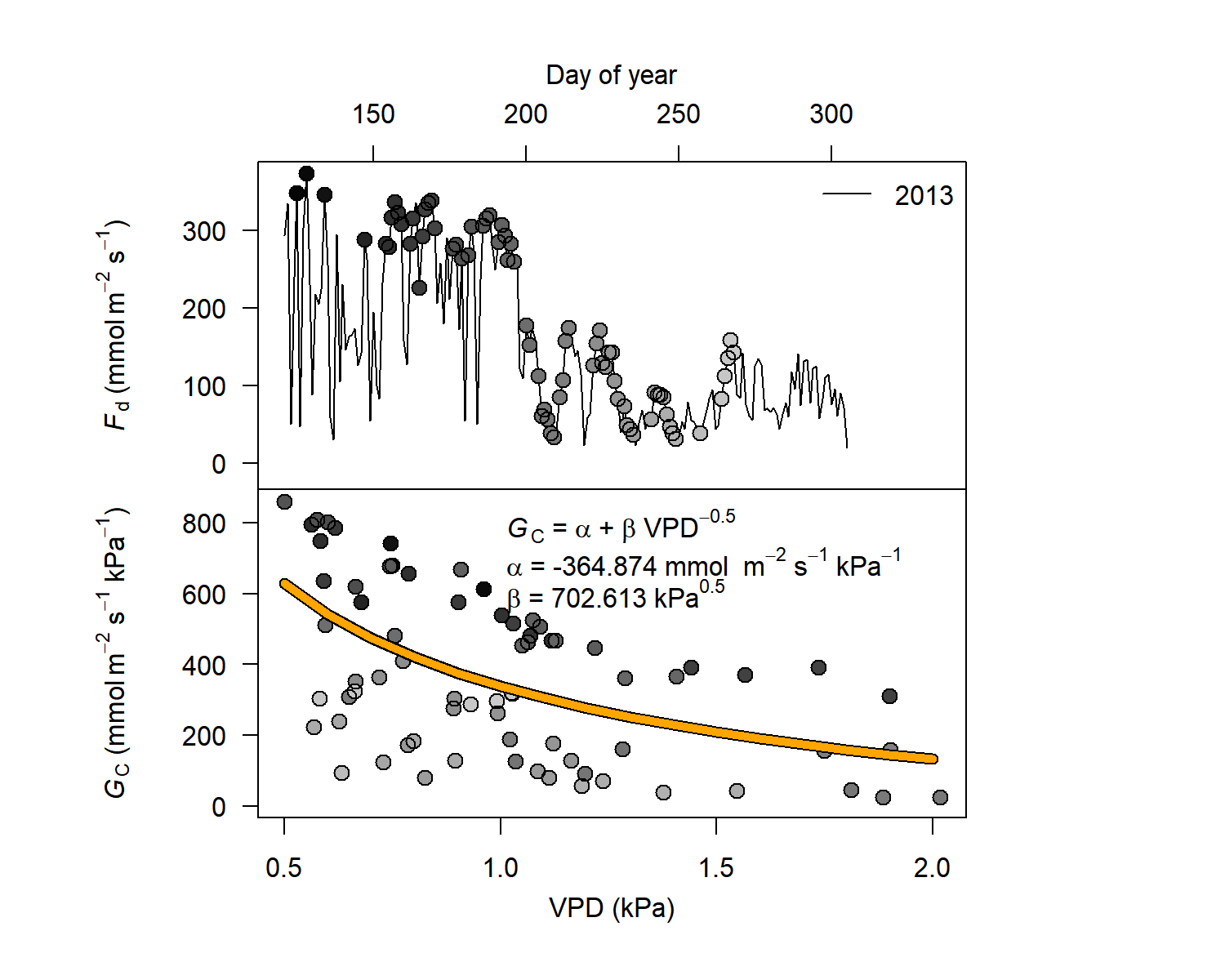TREX allows to assimilate, process and analyse sap flow data obtained with the thermal dissipation method (TDM). The package includes functions for gap filling time-series data, detecting outliers, calculating data-processing uncertainties and generating uniform data output and visualisation. The package is designed to deal with large quantities of data and apply commonly used data-processing methods. The functions have been validated on data collected from different tree species across the northern hemisphere (Peters et al. 2018 <doi: 10.1111/nph.15241>), and an accompanying manuscript has been published in Methods in Ecology and Evolution as (Peters et al. 2020 <doi: 10.1111/2041-210X.13524>)

## 1. Installation

The latest version of TREX can be installed and used via

remotes::install_github("the-Hull/TREX")

library(TREX)

If you want to use CRAN, we have a stable release version used for the MEE manuscript available named TREXr:

install.packages("TREXr")

## 2. Basic use and workflow

### Load data

# load raw data
raw   <- is.trex(example.data(type="doy"),
tz="GMT",
time.format="%H:%M",
solar.time=TRUE,
long.deg=7.7459,
ref.add=FALSE)

# adjust time steps
input <- dt.steps(input=raw,
start="2013-05-01 00:00",
end="2013-11-01 00:00",
time.int=15,
max.gap=60,
decimals=10,
df=FALSE)

# remove obvious outliers
input[which(input<0.2)]<- NA


### Calculate maximum ΔT-Values

Three methods can be applied to calculate ΔT (or ΔV for voltage differences between TDM probes):

• pd: pre-dawn
• mw: moving-window
• dr: double-regression
input <- tdm_dt.max(input,
methods = c("pd", "mw", "dr"),
det.pd = TRUE,
interpolate = FALSE,
max.days = 10,
df = FALSE)

plot(input$input, ylab = expression(Delta*italic("V"))) lines(input$max.pd, col = "green")
lines(input$max.mw, col = "blue") lines(input$max.dr, col = "orange")### Calculate Sap Flux Density


output.data<- tdm_cal.sfd(input,make.plot=TRUE,df=FALSE,wood="Coniferous")

plot(output.data$sfd.pd$sfd[1:1000, ], ylim=c(0,10))
# see estimated uncertainty
lines(output.data$sfd.pd$q025[1:1000, ], lty=1,col="grey")
lines(output.data$sfd.pd$q975[1:1000, ], lty=1,col="grey")
lines(output.data$sfd.pd$sfd[1:1000, ])

sfd_data <- output.data$sfd.dr$sfd### Generate Outputs

Here we generate outputs based on environmental filters and calculate crown conductance (Gc) values.

output<- out.data(input=sfd_data,
vpd.input=vpd,
sr.input=sr,
prec.input=preci,
low.sr = 150,
peak.sr=300,
vpd.cutoff= 0.5,
prec.lim=1,
method="env.filt",
max.quant=0.99,
make.plot=TRUE)## 3. More on TREX

### Workshops using TREX

• ESA 2020: TREX was introduced and demonstrated in detail in a workshop during the Ecological Society of America’s 2020 AGM. The workshop description can be found here, and all materials on the dedicated page.

## 4. Citing this work

Please cite TREX when you apply it in your own work as:

Peters, RL, Pappas, C, Hurley, AG, et al. Assimilate, process and analyse thermal dissipation sap flow data using the TREX r package. Methods Ecol Evol. 2021; 12: 342– 350. https://doi.org/10.1111/2041-210X.13524

A reference is available in R using:


citation("TREX")

#or

citation("TREXr")